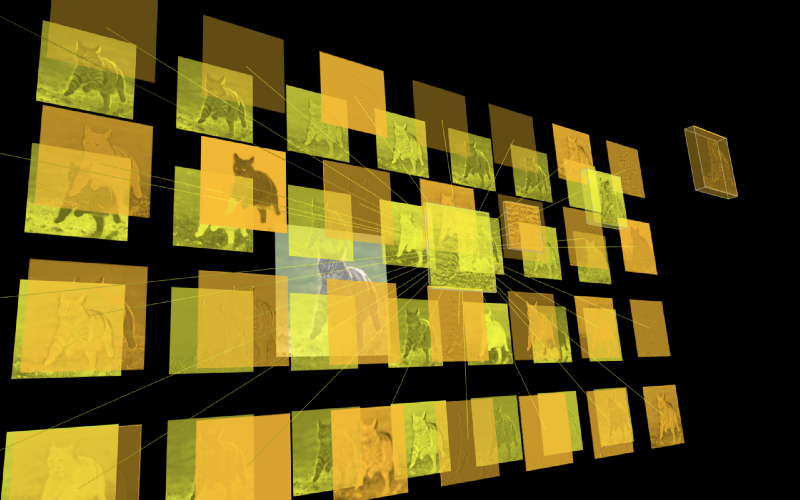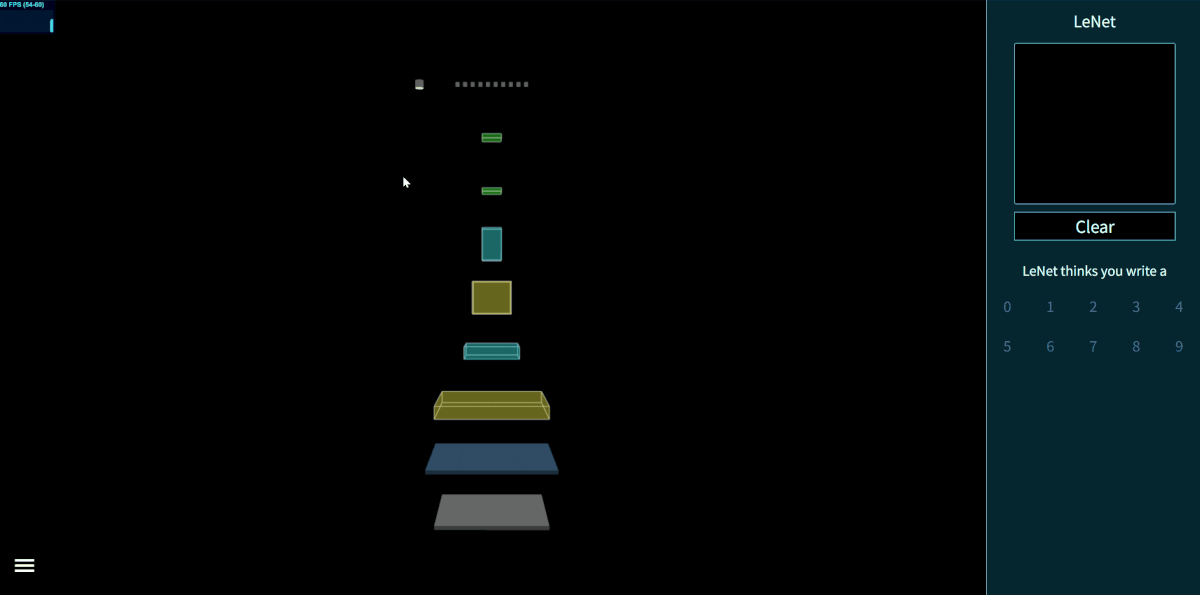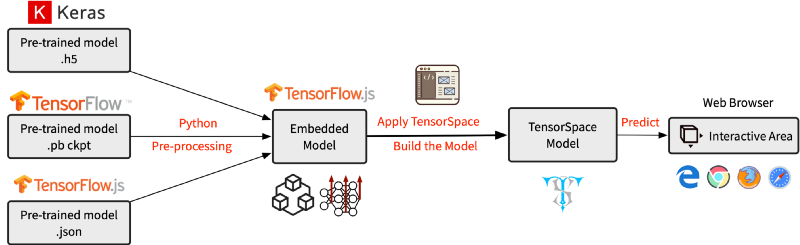by Chenhua Zhu

# Introducing TensorSpace.js — A Way to 3D Visualize Neural Networks in BrowsersFeature Abstractions of a Tiger Cat Image

Neural networks were always something high-level, unreachable and mysterious before I took my first deep learning class. To me they were just magic: neural network applications could complete tasks on object detection, image classification and even data prediction in our daily lives.

“What does the model compute?” “Why should we use this specific network for this task?” “How could others come up with a structure like this?”

Maybe you have the same questions as I had. My friends and I have found that sometimes it is really hard to understand and explain neural networks. Then we came up with some ideas:

Why not visualize a neural network?
How about a 3D model?
It can be interactive!

Since no such thing existed that we could find, we thought, why not create one ourselves? After 6 months, I am proud to introduce our effort: TensorSpace.js.

### What is TensorSpace.js?Fig. 1 — Interactive LeNet model created by TensorSpace.js
TensorSpace.js is a neural network 3D visualization framework built with TensorFlow.js, Three.js and Tween.js.

Since we wanted to be able to easily present the models in most web browsers, we choose JavaScript to implement the framework.

From Fig. 1 above, you can easily check out the model structure: each “cube” represents a “layer” object in the neural network.

Next, you can actually interact with the model by clicking, dragging and scrolling. Different angles may provide different view points of the model. Some objects are expandable, which allows you to observe more details.

Furthermore, we designed a hybrid architecture for TensorSpace.js to support different libraries, such as TensorFlow, Keras, and TensorFlow.js (more to come in the future).

TensorSpace.js can not only show the basic model structure, but also present the processes of internal feature abstractions, intermediate data manipulations, and final inference generations.

In summary, TensorSpace.js is:

• Interactive — Uses Keras-like API to build interactive models in browsers.
• Intuitive — Visualizes the information from intermediate inferences.
• Integrative — Supports pre-trained models from TensorFlow, Keras, and TensorFlow.js.

### How does TensorSpace.js work?

The following part introduces how to build a TensorSpace model. I’m using an LeNet handwritten recognition model as an example. You can find all the example files from the repo here: TensorSpace — HelloWorld.Fig. 2 — Workflow to present a TensorSpace model

The general workflow is to create or preprocess a pre-trained model with multiple intermediate outputs. Then, based on the neural network structure, we can build a TensorSpace model. Last, we load and initialize the model which can accept input data for inferences.

After correctly installing TensorSpace.js and properly preprocessing the pre-trained models, we can easily create an LeNet handwritten recognition TensorSpace model. For convenience, we use the preprocessed TensorSpace compatible model and an extracted file which is a handwritten “5” as the model input.

``let container = document.getElementById( "container" );``
``// Create sequential modellet model = new TSP.models.Sequential( container );``
``// Add LeNet Layersmodel.add( new TSP.layers.GreyscaleInput({ shape: [28, 28, 1] }) );model.add( new TSP.layers.Padding2d({ padding: [2, 2] }) );model.add( new TSP.layers.Conv2d({ kernelSize: 5, filters: 6, strides: 1 }) );model.add( new TSP.layers.Pooling2d({ poolSize: [2, 2], strides: [2, 2] }) );model.add( new TSP.layers.Conv2d({ kernelSize: 5, filters: 16, strides: 1 }) );model.add( new TSP.layers.Pooling2d({ poolSize: [2, 2], strides: [2, 2] }) );model.add( new TSP.layers.Dense({ units: 120 }) );model.add( new TSP.layers.Dense({ units: 84 }) );model.add( new TSP.layers.Output1d({    units: 10,    outputs: ["0", "1", "2", "3", "4", "5", "6", "7", "8", "9"]}) );``
``// Load preprocessed modelmodel.load({    type: "tfjs",    url: './lenetModel/mnist.json'});``
``// Initialize the model in the browsermodel.init(function() {    // Predict input "5"    model.predict( image_5 ); });``

That’s it!

You can try it out in the CodePen:

It is easy to build other preprocessed models in the same way. If you are interested, please check out our playground for more interesting demos, such as Yolov2-tiny, ACGAN, and ResNet-50.